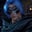Related Tags

typescript
communitycreator

# What are numbers in TypeScript?Gutha Vamsi Krishna

In TypeScript, number is a data type to represent data like 1, 1.5, 0b111001, etc.

• number is stored as a Number object, where Number is a wrapper class to manipulate numbers as objects.
• It doesn’t have different data types, like int, float, or double. Everything is simply a number in TypeScript.

### Syntax

var variable_name:number = value


### Code

The following example shows the usage of data type number. Here, y is the variable name, number is the data type, and 5 is the value.

var y:number = 5
console.log(y)

The following code snippet shows that we can assign float, binary, and hexadecimal values to the number datatype.

// representing floating point numbers
var f:number = 1.2
console.log(f)

//representing decimal numbers
var b:number  = 0b111001
console.log(b)

var h:number = 0x37CF
console.log(h)

### Operations on numbers

We can perform many math operations on numbers, like addition, subtraction, multiplication, etc.

The following code snippet shows the usage of some of the operators.

var x:number = 10
var y:number = 20

console.log(Addition  = ${x+y}) //subtraction console.log(Subtraction =${y-x})

//Multiplication
console.log(Multiplication  = \${x*y})

### Number methods

The Number wrapper provides the following methods on numbers:

• toExponential()
• toFixed()
• toLocaleString()
• toPrecision()
• toString()
• valueOf()

We will go through each of them in detail.

#### toExponential()

This method returns the exponential notation of a given number in string format. It accepts one optional parameter to specify the number of digits to be placed after the decimal point.

var x:number = 1357

//without any parameter
console.log(x.toExponential())

//with 1 as parameter, one digit will present after decimal point
console.log(x.toExponential(1))

#### toFixed()

This method returns a fixed-point notation of the given number in string format. It accepts one optional parameter to specify the number of digits after the decimal point.

var x:number = 20.8938

//without paramters, it will be fixed to 21
console.log(x.toFixed())

//2 as parameter, so 2 digits will present after decimal
console.log(x.toFixed(2))

#### toLocaleString()

This method will return the given number in local string format. Please try to execute the following code snippet in TypeScript playground.

let x: number = 11677.123;

//returns "11,677.123"  in US English format
console.log(x.toLocaleString());

// returns "11.677,123" in German
console.log(x.toLocaleString('de-DE'));

// returns "١١٬٦٧٧٫١٢٣"  in Arabic format
console.log(x.toLocaleString('ar-EG'));

#### toPrecision()

This method returns a fixed-point or exponential format for the given number. It accepts an optional parameter to specify the number of digits for precision.

var x:number = 11.5987

// 1 as paramter to specify precision to 1 digit
console.log(x.toPrecision(1))

// 2 as paramter to specify precision to 2 digits
console.log(x.toPrecision(2))

#### toString()

This method returns the number in string format for a given base.

The base must be between $2$ and $36$.

var x:number = 987
//converting 987 into base 2 format
console.log(x.toString(2))

#### valueOf()

This method returns the primitive type of the given object. We can create a number object with the Number class.

//constructing number object
var x = new Number(20)
//printing primitive value of object x
console.log(x.valueOf())

RELATED TAGS

typescript
communitycreator

CONTRIBUTORGutha Vamsi Krishna
RELATED COURSES

View all Courses

Keep Exploring

Learn in-demand tech skills in half the time